Submit a blog to KDnuggets -- Top Blogs Win A Reward

Topics: AI | Data Science | Data Visualization | Deep Learning | Machine Learning | NLP | Python | R | Statistics

KDnuggets Home » News » 2017 » Aug » Opinions, Interviews » DeepMind Relational Reasoning Networks  Demystified ( 17:n31 )

#DeepMind Relational Reasoning Networks  Demystified

http likes 178

Every time DeepMind publishes a new paper, there is frenzied media coverage around it. We examine what is and is not real in recent work described as “DeepMind Neural Network Can Make Sense of Objects Around It”.comments

Every time DeepMind publishes a new paper, there is frenzied media coverage around it. Often you will read phrases that are often misleading. For example, its new paper on relational reasoning networks has futurism reporting it like

DeepMind Develops a Neural Network That Can Make Sense of Objects Around It.

This is not only misleading, but it also makes the everyday non PhD person intimidated. In this post I will go through the paper in an attempt to explain this new architecture in simple terms.

You can find the original paper here.

I will follow the paper’s structure as much as possible. I will add my own bits to simply the material.

### What is Relational Reasoning?

In its simplest form, Relational Reasoning is learning to understand relations between different objects(ideas). This is considered an essential characteristic of intelligence. The authors have included a helpful infographic to explain what it is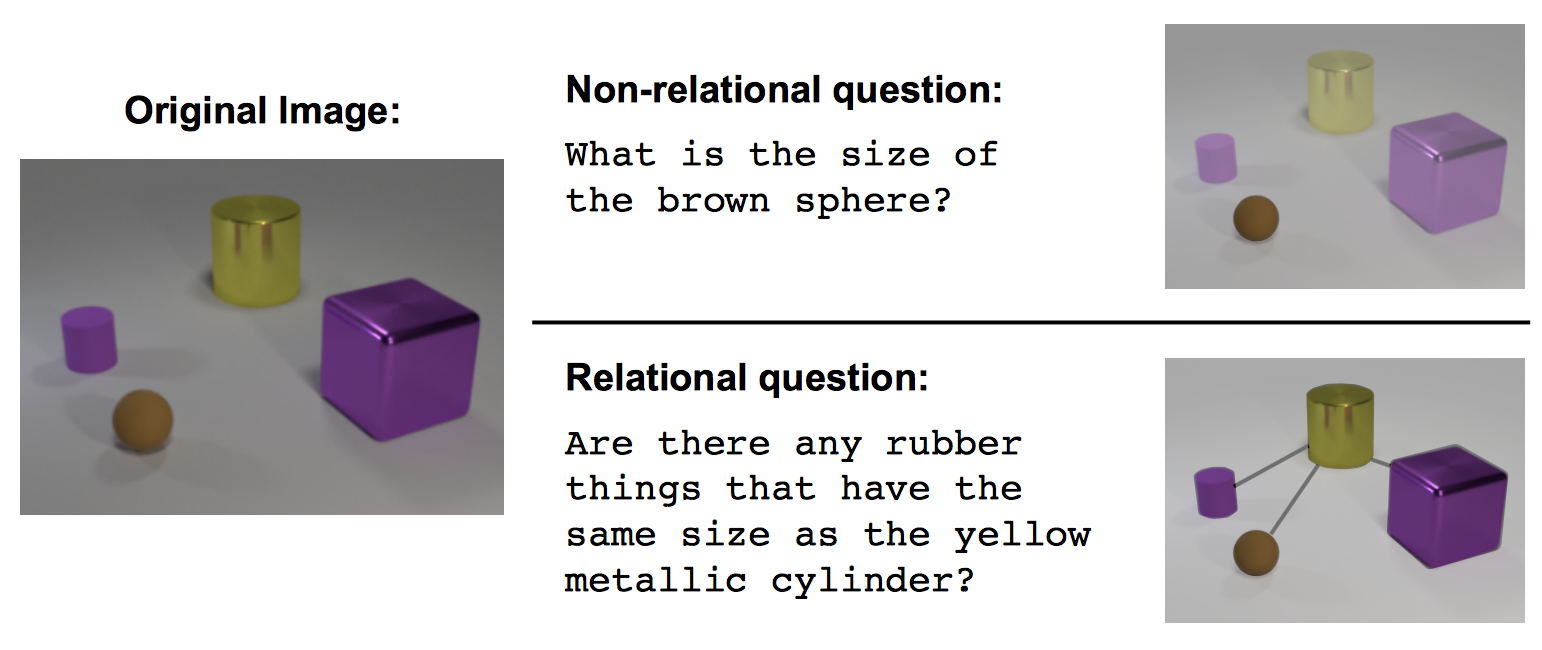Figure1.0 The model has to look at objects of different shape/size/color, and be able to answer questions that are related between multiple such objects.

### Relational Networks

The authors have presented a neural network that is made to inherently capture relations(e.g. Convolutional Neural networks are made to capture properties of images). They presented an architecture that is defined like so :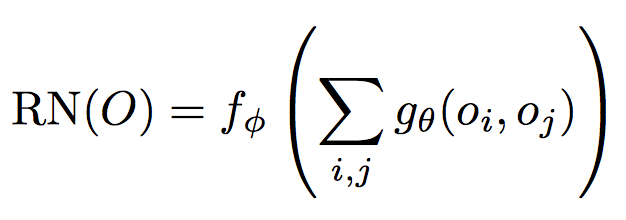Equation1.0 Definition of Relational Networks

#### Explained

The Relational Network for O (O is the set of objects you want to learn relations of) is a function fɸ.

gθ is another function that takes two objects :oi , and oj. The output of gθ is the ‘relation’ that we are concerned about.

Σ i,j means , calculate gθ for all possible pairs of objects, and then sum them up.

### Neural Networks and Functions

It is easy to forget this when learning about neural networks, backprop ,etc. but a neural network is in fact a single mathematical function! Therefore, the function that I described in Equation 1.0 is a neural network!. More precisely , there are two neural networks:

1. gθ, which calculates relations between a pair of objects
2. fɸ, which takes in the sum of all gθ, and calculates the final output of the model

Both  , and  are multi layer perceptrons in the simplest case.

### Relational Neural Networks are flexible

The authors present Relational Neural Network as a module. It can accept encoded objects and learn relations from them, but more importantly, they can be plugged into Convolutional Neural networks , and LSTMs.

The Convolutional network can be used to learn the objects using images. This makes it far more useful for applications because reasoning on an image is more useful than reasoning on an array of user defined objects.

The LSTMs along with word embeddings can be used to understand the meaning of the query that the model has been asked. This is again , more useful because the model can now accept an English sentence instead of encoded arrays.

The authors have presented a way to combine relational networks, convolutional networks , and LSTMs to construct an end to end neural network that can learn relations between objects.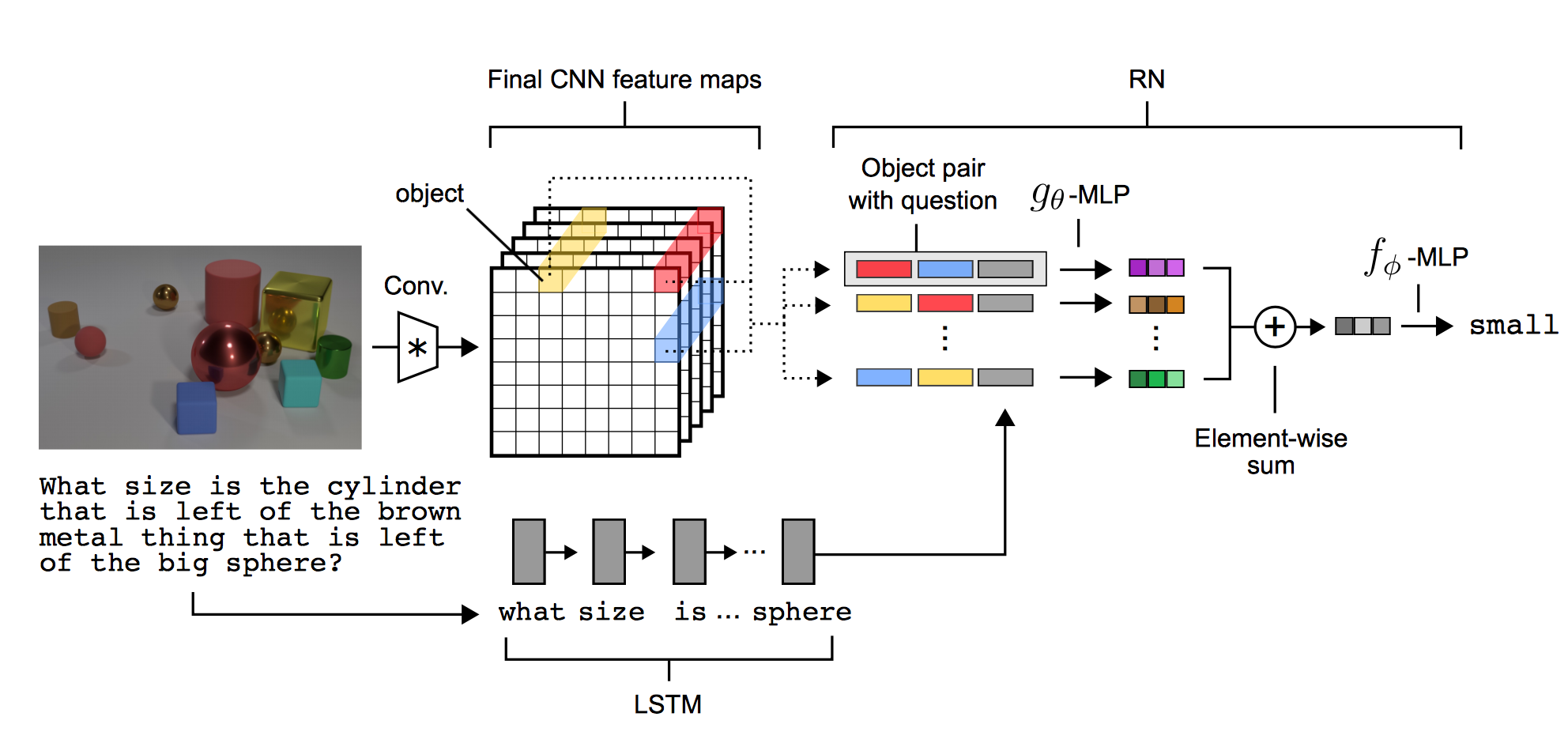Figure 2.0 An end to end relational reasoning neural network.

### Figure 2.0 Explanation

The image is passed through a standard Convolutional Neural network(CNN), which can extract features of that image in k filters. The ‘object’ for the relational network is a vector of features of each point in the grid. e.g. one ‘object’ is the yellow vector.

The question is passed through an LSTM , which produces a feature vector of that question. This is roughly the ‘idea’ of that question.

This modifies the original Equation 1.0 slightly. It adds another term which makes it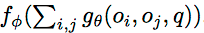Notice the extra q in Equation 1.0. That q is the final state of the LSTM. The relations are now conditioned using q.

After that, the ‘object’ from the CNN and the vector from the LSTM are used to train the relational network. Each object pair is taken, along with the question vector from the LSTM, and those are used as inputs for gθ(which is a neural network).

The outputs of gθ are then summed up , and used as inputs to fɸ(which is another neural network). fɸ is then optimsed on the answer to the question.

### Benchmarks

The authors demonstrate the effectiveness of this model on several datasets. I will go through one of them (and in my opinion the most notable) — CLEVR dataset.

The CLEVR dataset consists of images of objects of different shapes,sizes and color. The model is asked questions about these images like:

Is the cube the same material as the cylinder?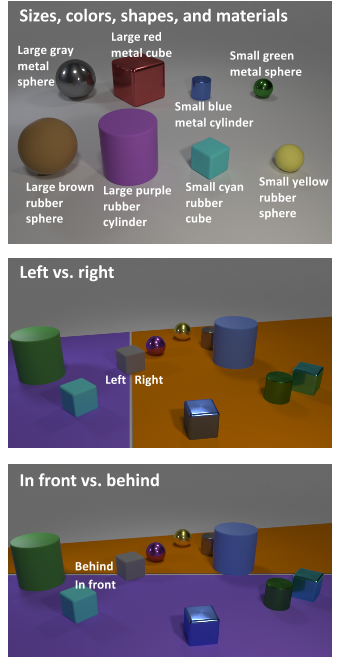The authors point out that other systems are far behind their own model in terms of accuracy. This is because Relational networks are designed to capture relations.

Their model achieves an unprecedented 96% + accuracy, as compared to a mere 75% (using stacked attention models)

Figure 3.0 The types of objects(top),and the positioning scheme (centre&bottom)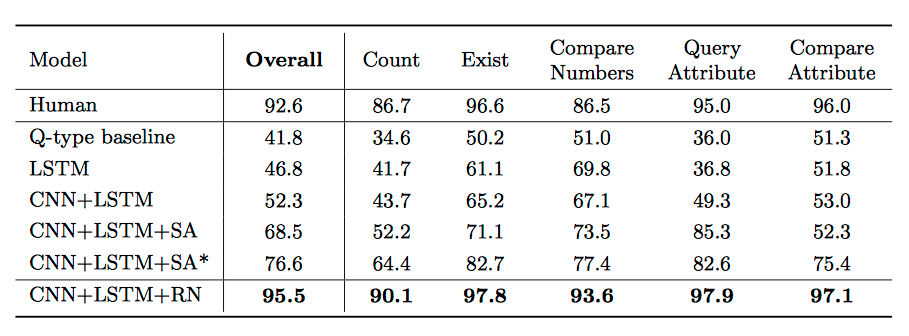Figure3.1 Comparison between different architectures on the CLEVR dataset using pixels(i.e. not matrix encoded)

### Conclusion

Relational Networks are extremely adept at learning relations. They do so in a data efficient manner. They are also flexible and can be used as a drop in solution when using CNN’s, LSTMs, or both.

This post was about debunking the ‘AI has taken over’ hype caused by very large publications, and giving some perspective on what the current state of the art is.

Original. Reposted with permission.

Related:

## Top Stories Past 30 Days

Most Popular
Most SharedGet KDnuggets, a leading newsletter on AI, Data Science, and Machine Learning